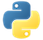# MinPydoc

Gets the element with the minimum value within each aggregation.

## Examples

In the following example, we create a pipeline with a `PCollection`. Then, we get the element with the minimum value in different ways.

### Example 1: Minimum element in a PCollection

We use `Combine.Globally()` to get the minimum element from the entire `PCollection`.

``````import apache_beam as beam

with beam.Pipeline() as pipeline:
min_element = (
pipeline
| 'Create numbers' >> beam.Create([3, 4, 1, 2])
| 'Get min value' >>
beam.CombineGlobally(lambda elements: min(elements or [-1]))
| beam.Map(print))``````

Output:

``1``View source code

### Example 2: Minimum elements for each key

We use `Combine.PerKey()` to get the minimum element for each unique key in a `PCollection` of key-values.

``````import apache_beam as beam

with beam.Pipeline() as pipeline:
elements_with_min_value_per_key = (
pipeline
| 'Create produce' >> beam.Create([
('🥕', 3),
('🥕', 2),
('🍆', 1),
('🍅', 4),
('🍅', 5),
('🍅', 3),
])
| 'Get min value per key' >> beam.CombinePerKey(min)
| beam.Map(print))``````

Output:

``````('🥕', 2)
('🍆', 1)
('🍅', 3)``````View source codePydoc